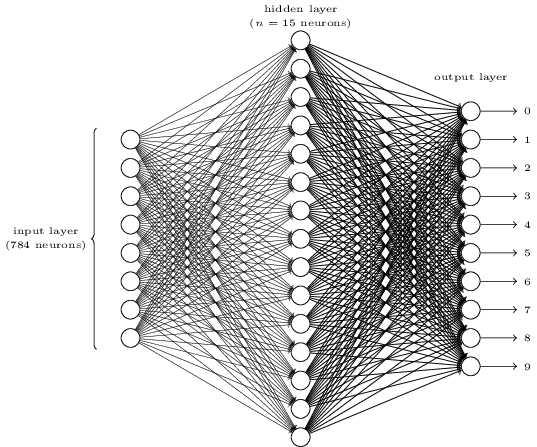# CS224N（1.24）Language Models and RNNs

\begin{eqnarray}P(x^{(t+1)}|x^{(t)},...,x^{(1)})\tag{1}\end{eqnarray}

$x^{(t+1)}$表示第$t+1$个位置（时刻）的词是$x$$x$可以是词典$V$中的任意一个词。

# CS224N（1.15 & 1.17）BackpropagationContinue reading